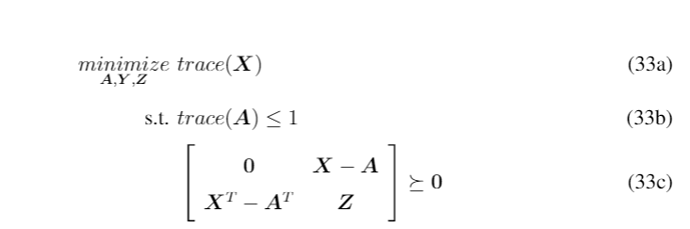# LMI constraint is falied

## Hello, Im solving problemimage732×243 8.19 KB Clearly, we have X==A due to the LMI constraint (33c). But i didnot get the wanted results when i run the matlab code M=8 cvx_begin sdp variable A(M+1,M+1) variable X(M+1,M+1) hermitian variable Z(M+1,M+1) hermitian [zeros(M+1,M+1) X-A; X’-(A)’ Z;]>=0 trace(A)<=1 maximize (trace(X) ) cvx_end

Status: Unbounded
Optimal value (cvx_optval): +Inf
Results show that Z is great, (1e6) and X-A is not small.

## Of course by adding constraint Trace(Z)<=100, CVX solved the problem and we get X-A=0 and the results is as follows

Status: Inaccurate/Solved
Optimal value (cvx_optval): +1.00573
But Adding this constraint is not good for my problem considering something else in my problem.

Is there any other way to solve such problem?
(33aq) says `minimize trace (X)`. Your program has `maximize(trace(X))` .The CVX result is correct, because (trace of) `X` and `Z` can increase together without limit while maintaining feasibility. it appears your program has a “typo” and should instead have `minimize(trace(X))`.